# SSAT Upper Level Math : How to find a ratio

## Example Questions

← Previous 1 2 Next →

### Example Question #1 : Decide If Two Quantiies Are In A Proportional Relationship: Ccss.Math.Content.7.Rp.A.2a

John and Michela are business partners who agreed to split profits at a ratio of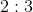, with Michela taking the larger share. If their business made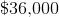in the first year, how much money did Michela make?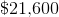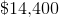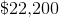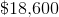Explanation:

Letbe the amount John takes home andbe the amount Michela takes home.

Since their business made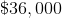,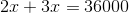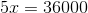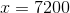We want to know how much Michela made so we need to find the value of.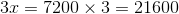### Example Question #3 : Decide If Two Quantiies Are In A Proportional Relationship: Ccss.Math.Content.7.Rp.A.2a

The angles in a triangle have a ratio of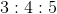. What is the measurement of the smallest angle?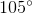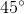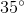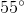Explanation:

Let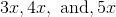be the values of the angles.

Since there aredegrees in a triangle,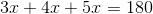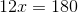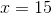Since we want the value of the smallest angle, find the value of.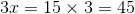### Example Question #11 : How To Find A Ratio

In a factory, there are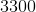glass bottles. If the ratio of red bottles to blue bottles is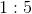, how many more blue bottles than red bottles are there?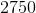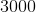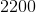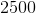Explanation:

First, find the number of red bottles and blue bottles.

Letbe the number of red bottles and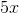be the number of blue bottles. Since there is a total ofbottles at the factory,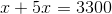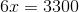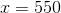There are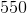red bottles. Find the value ofto find the number of blue bottles.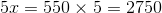Now, because the question wants to find how many more blue bottles than red bottles there are, subtract the number of red bottles from the number of blue bottles.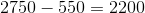### Example Question #11 : Rational Numbers

The ratio of offensive players to defensive players on a football team is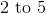. If there areplayers on the team, how many offensive players are there?Explanation:

Letbe the number of offensive players andbe the number of defensive players.

Since there is a total ofplayers on the team,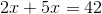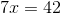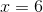We need to find the number of offensive players, so we will need to find the value of.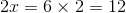### Example Question #13 : How To Find A Ratio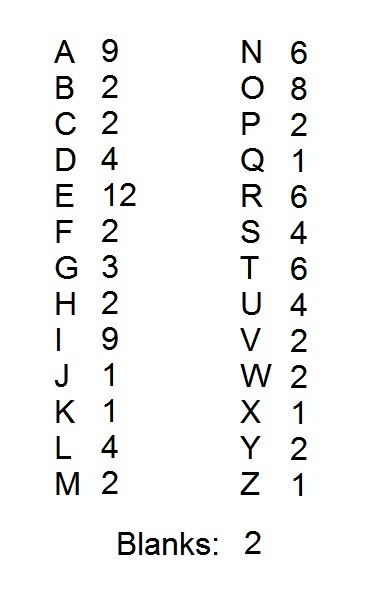A popular word game uses one hundred tiles, each of which is marked with a letter or a blank. The distribution of the tiles is shown above, with each letter paired with the number of tiles marked with that letter. Notice that there are two blank tiles.

If the tiles marked with an "E" are removed, and the rest are placed in a box, then what is the ratio of consonant tiles to vowel tiles in the box?

Note: for purposes of this problem, "Y" is considered a consonant.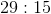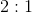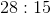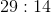Explanation:

Out of the 100 tiles, there are nine "A" tiles, twelve "E" tiles, nine "I" tiles, eight "O" tiles, and four "U" tiles.

If the "E" tiles are removed, there will be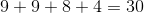vowel tiles.

The number of consonant tiles can most easily be found by adding the number of vowel tiles and blanks: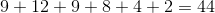.

The rest of the tiles are consonant tiles; subtract from 100 to get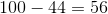of them.

Therefore, the ratio of consonant tiles to vowel tiles in the box after removing the "E's" is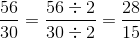- that is, a 28 to 15 ratio.

← Previous 1 2 Next →

### All SSAT Upper Level Math Resources